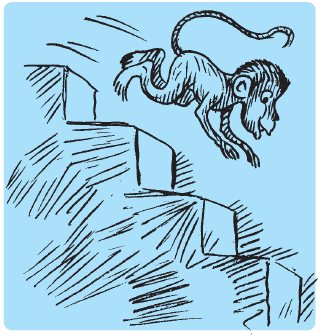# A water tank has steps inside it. A monkey is sitting on the topmost step (i.e., the first step). The water level is at the ninth step. (i) He jumps 3 steps down and then jumps back 2 steps up. In how many jumps will he reach the water level? (ii) After drinking water, he wants to go back. For this, he jumps 4 steps up and then jumps back 2 steps down in every move. In how many jumps will he reach back the top step? ](ii) After drinking water, he wants to go back. For this, he jumps 4 steps up and then jumps back 2 steps down in every move. In how many jumps will he reach back the top step?Let us assume that the steps moved down be denoted by positive integers.

The steps moved up be denoted by negative integers.

(i) Originally, the monkey was at step = 1

After 1st jump, the monkey will be at step = 1 + 3 = 4

After 2nd jump, the monkey will be at step = 4 + (−2) = 2

After 3rd jump, the monkey will be at step = 2 + 3 = 5

After 4th jump, the monkey will be at step = 5 + (−2) = 3

After 5th jump, the monkey will be at step = 3 + 3 = 6

After 6th jump, the monkey will be at step = 6 + (−2) = 4

After 7th jump, the monkey will be at step = 4 + 3 = 7

After 8th jump, the monkey will be at step = 7 + (−2) = 5

After 9th jump, the monkey will be at step = 5 + 3 = 8

After 10th jump, the monkey will be at step = 8 + (−2) = 6

After 11th jump, the monkey will be at step = 6 + 3 = 9

Therefore, the monkey will be at water level (i.e., 9th step) after 11 jumps.

(ii)At first, the monkey was at step = 9

After 1st jump, the monkey will be at step = 9 + (−4) = 5

After 2nd jump, the monkey will be at step = 5 + 2 = 7

After 3rd jump, the monkey will be at step = 7 + (− 4) = 3

After 4th jump, the monkey will be at step = 3 + 2 = 5

After 5th jump, the monkey will be at step = 5 + (− 4) = 1

Clearly, the monkey will reach back at the top step after 5 jumps.

(iii) If steps moved down are denoted by negative integers

The steps moved up are denoted by positive integers, then his moves will be as follows.

Moves in part (i)

− 3 + 2 − 3 + 2 − 3 + 2 − 3 + 2 − 3 + 2 − 3 = −8

Moves in part (ii)

4 − 2 + 4 − 2 + 4 = 8

Moves in part (ii) denotes going up 8 steps.(0)(0)